# HSSlive: Plus One & Plus Two Notes & Solutions for Kerala State Board

## AP Board Class 7 Maths Chapter 13 Area and Perimeter InText Questions Textbook Solutions PDF: Download Andhra Pradesh Board STD 7th Maths Chapter 13 Area and Perimeter InText Questions Book AnswersAP Board Class 7 Maths Chapter 13 Area and Perimeter InText Questions Textbook Solutions PDF: Download Andhra Pradesh Board STD 7th Maths Chapter 13 Area and Perimeter InText Questions Book Answers

## Andhra Pradesh State Board Class 7th Maths Chapter 13 Area and Perimeter InText Questions Books Solutions

 Board AP Board Materials Textbook Solutions/Guide Format DOC/PDF Class 7th Subject Maths Chapters Maths Chapter 13 Area and Perimeter InText Questions Provider Hsslive

2. Click on the Andhra Pradesh Board Class 7th Maths Chapter 13 Area and Perimeter InText Questions Answers.
3. Look for your Andhra Pradesh Board STD 7th Maths Chapter 13 Area and Perimeter InText Questions Textbooks PDF.
4. Now download or read the Andhra Pradesh Board Class 7th Maths Chapter 13 Area and Perimeter InText Questions Textbook Solutions for PDF Free.

## AP Board Class 7th Maths Chapter 13 Area and Perimeter InText Questions Textbooks Solutions with Answer PDF Download

Find below the list of all AP Board Class 7th Maths Chapter 13 Area and Perimeter InText Questions Textbook Solutions for PDF’s for you to download and prepare for the upcoming exams:

Try This

Question 1.
ABCD is a parallelogram with sides 8 cm and 6 cm. In Figure 1, what is the base of the parallelogram? What is the height? What is the area of the parallelogram? In Figure 2, what is the base of the parallelogram? What is the height? What is the area of the parallelogram? Is the area of Figure 1 and Figure 2 the same ? (Page No. 248)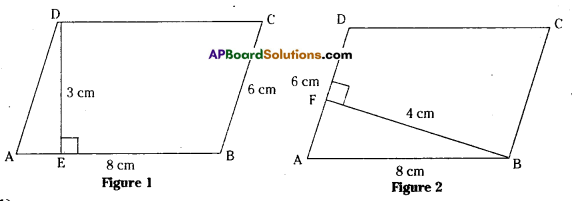Solution:
In fig (1)
Base of the parallelogram ABCD is 8cm.
Side of the parallelogram ABCD is 6cm.
Height of the parallelogram ABCD is 3cm.
Area of the parallelogram ABCD = base x height = 8×3 = 24cm2
In fig (2)
Base of the parallelogram = 6cm
Side of the.parallelogram = 8cm
Height of the parallelogram = 4cm
Area of the parallelogram = base x height = 6×4 = 24cm2
Yes. Areas of fig (1) & fig (2) are equal.

Do This

Question 1.
In parallelogram ABCD, AB = 10 cm and DE = 4cm. Find (i) the area of ABCD ii) the length of BF, if AD = 6cm (Page No. 248)
Solution:
Given AB = 10 cm, DE = 4 cm
Area of parallelogram ABCD = Base x Height
= 10 x 4 = 40 cm2
Also area of parallelogram ABCD = Base x Height
40 = 6 x BF
BF = 406=203=623= 6.6cm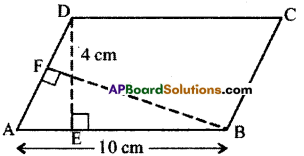Do This

Question 1.
Carefully study the following parallelogram. (Page No. 248)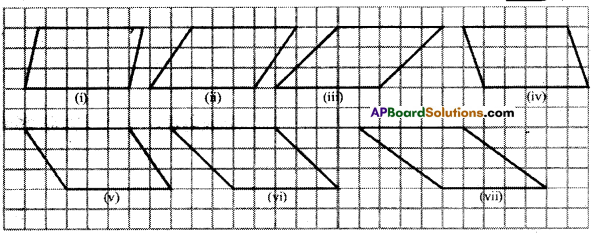i) Find the area of the each parallelogram by counting the squares enclosed in it. For counting incomplete squares check whether two incomplete squares make a complete square in each parallelogram. Complete the following table accordingly.
Solution: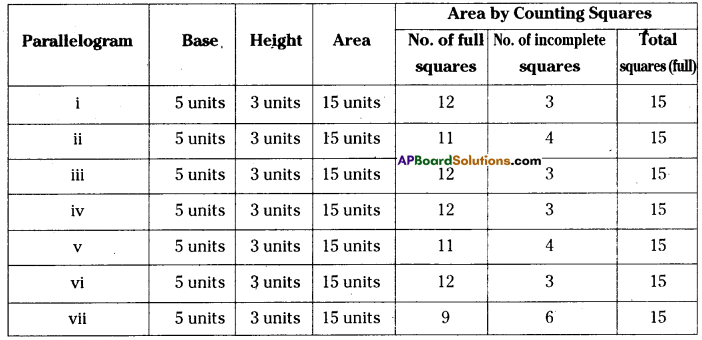(ii) Do all parallelograms with equal base and equal heights have the same area ?
Solution:
Yes

Try This

Question

i) Why is the formula for finding the area of a rectangle related to the formula for finding the area of a parallelogram ? (Page No. 249)
Solution:
Any parallelogram can be modelled into a rectangle and hence, area of a parallelogram is related to the area of a rectangle.

ii) Explain why a rectangle is a parallelogram but a parallelogram may not be a rectangle.
Solution:
In a rectangle both pairs of opposite sides are parallel and hence it is a parallelogram. But each angle of a parallelogram is not always a right angle and hence it may not be a rectangle.

Try This

Question 1.
In the figure all triangles are on the base AB = 24 cm. Is the height of each of the triangles drawn on base AB, the same ? Will all the triangles have equal area ? Give reasons to support your answer. Are the triangles congruent also ? (Page No. 252)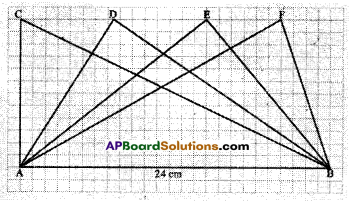Solution:
Heights of all triangles are equal, as the triangles are drawn between same parallels.
Areas of all triangles are equal, as all the triangles can be modelled as half of same parallelo¬gram. But triangles are not congruent.

## Andhra Pradesh Board Class 7th Maths Chapter 13 Area and Perimeter InText Questions Textbooks for Exam Preparations

Andhra Pradesh Board Class 7th Maths Chapter 13 Area and Perimeter InText Questions Textbook Solutions can be of great help in your Andhra Pradesh Board Class 7th Maths Chapter 13 Area and Perimeter InText Questions exam preparation. The AP Board STD 7th Maths Chapter 13 Area and Perimeter InText Questions Textbooks study material, used with the English medium textbooks, can help you complete the entire Class 7th Maths Chapter 13 Area and Perimeter InText Questions Books State Board syllabus with maximum efficiency.

## FAQs Regarding Andhra Pradesh Board Class 7th Maths Chapter 13 Area and Perimeter InText Questions Textbook Solutions

#### Can we get a Andhra Pradesh State Board Book PDF for all Classes?

Yes you can get Andhra Pradesh Board Text Book PDF for all classes using the links provided in the above article.

## Important Terms

Andhra Pradesh Board Class 7th Maths Chapter 13 Area and Perimeter InText Questions, AP Board Class 7th Maths Chapter 13 Area and Perimeter InText Questions Textbooks, Andhra Pradesh State Board Class 7th Maths Chapter 13 Area and Perimeter InText Questions, Andhra Pradesh State Board Class 7th Maths Chapter 13 Area and Perimeter InText Questions Textbook solutions, AP Board Class 7th Maths Chapter 13 Area and Perimeter InText Questions Textbooks Solutions, Andhra Pradesh Board STD 7th Maths Chapter 13 Area and Perimeter InText Questions, AP Board STD 7th Maths Chapter 13 Area and Perimeter InText Questions Textbooks, Andhra Pradesh State Board STD 7th Maths Chapter 13 Area and Perimeter InText Questions, Andhra Pradesh State Board STD 7th Maths Chapter 13 Area and Perimeter InText Questions Textbook solutions, AP Board STD 7th Maths Chapter 13 Area and Perimeter InText Questions Textbooks Solutions,
Share: